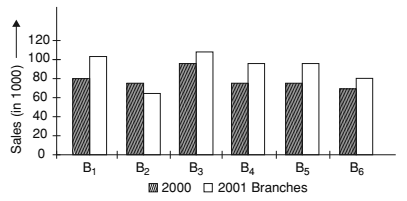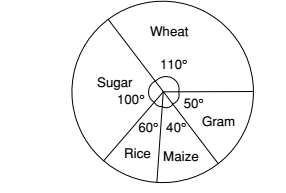## Introduction to Data Interpretation

#### Data Interpretation

Direction: Bar chart showing the sales of books (in 1000) from six branches B1, B2, B3, B4, B5 and B6 of a publishing company in 2000 and 2001 is given below. Study the chart and answer the questions.1. Total sales of Branch B6 for both the years is what per cent of the total sales of Branch B3 for both the years?

1.  ∴ Required Percentage = Total sales of Branch B6 in 2000 and 2001 years x 100 % Total sales of Branch B3 in 2000 and 2001 years

##### Correct Option: B

 ∴ Required Percentage = Total sales of Branch B6 in 2000 and 2001 years x 100 % Total sales of Branch B3 in 2000 and 2001 years

Total sales of Branch B6 in 2000 and 2001 years = 70 + 80 = 150
Total sales of Branch B3 in 2000 and 2001 years = 95 + 110 = 205
 Required Percentage = 150 x 100 = 73.17% 205

1. What is the ratio of the total sales of Branch B2 for both the years to the total sales of Branch B4 for both the years?

1. ∴ Required ratio = the ratio of the total sales of Branch B2 in 2000 and 2001 years : the ratio of the total sales of Branch B4 in 2000 and 2001 years

##### Correct Option: D

∴ Required ratio = the ratio of the total sales of Branch B2 in 2000 and 2001 years : the ratio of the total sales of Branch B4 in 2000 and 2001 years
the ratio of the total sales of Branch B2 in 2000 and 2001 years = 75 + 65 = 140
the ratio of the total sales of Branch B4 in 2000 and 2001 years = 85 + 95 = 180
Required ratio = 140:180 = 7:9

1. What percent of the average sales of branches B1, B2 and B3 in 2001 is the average sales of branches B1, B3 and B6in 2000?

1.  ∴ Required percentage = Average sales of branches B1, B2 and B3 in 2001 x 100% Average sales of branches B1, B3 and B6in 2000

##### Correct Option: D

Average sales of branches B1, B2 and B3 in 2001

 = 105 + 65 + 110 = 280 3 3

Average sales of branches B1, B3 and B6 in 2000
 = 80 + 95 + 70 = 245 3 3

 ∴ Required percentage = Average sales of branches B1, B2 and B3 in 2001 x 100% Average sales of branches B1, B3 and B6in 2000

 ∴ Required percentage = 280/3 x 100 = 114.28 245/3

1. What is the average sale of books from all the branches for the year 2000?

1.  Required average = B1 + B2 + B3 + B4 + B5 + B6 6

##### Correct Option: B

 Required average = B1 + B2 + B3 + B4 + B5 + B6 6

Required average
 = 80 + 75 + 95 + 85 + 75 + 70 = 480 = 80 6 6

Direction: The annual agricultural production (in tonnes) of an Indian state is given in the pie chart. The total production is 9000 tonnes. Read the pie chart and answer the question.1. What is the annual production of wheat?

1. Given :- 360° = 9000 tonnes

 then ,1° = 9000 tonnes 360

##### Correct Option: A

Given :- 360° = 9000 tonnes

 then ,1° = 9000 tonnes 360

 ∴ the annual production of wheat = 110° ≡ 9000 x 110 = 2750 tonnes 360